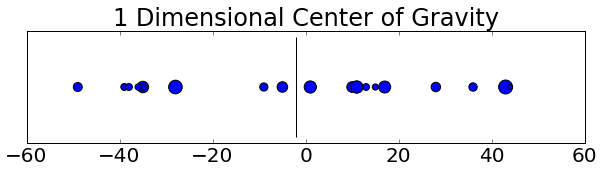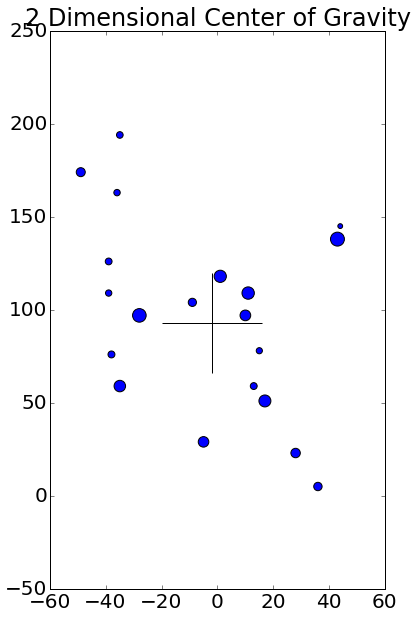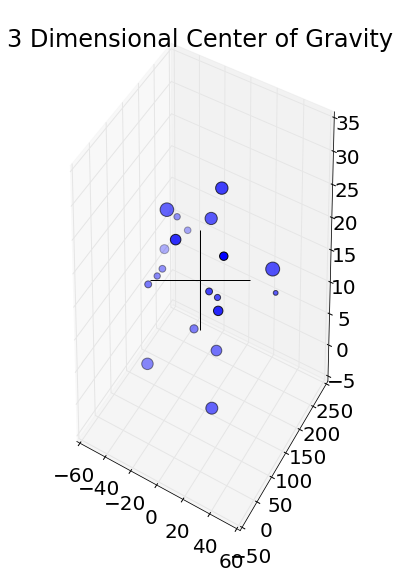﻿ Finding the Center of Mass from Point Masses with Python - if curious: then learn

# Finding the Center of Gravity(CG) of a point mass in 1, 2 and 3 Dimensions¶

First, import python modules and set up the plotting

In :
import numpy as np
import matplotlib.pyplot as plt
from mpl_toolkits.mplot3d import Axes3D


When using ipython notebooks, plotting inline can be achieved by execute the matplotlib magic function

In :
%matplotlib inline

In :
#set plot sizes
plt.rcParams['figure.figsize'] = (10, 2)  # (width, height)
plt.rcParams['font.size'] = 20
plt.rcParams['legend.fontsize'] = 16


## First, lets determine the CG of a 1D space¶

Lets randomly assign an x coordinate value for the point mass from [-50, 50] with n=20

In :
n=20
x = np.random.randint(-50, 50, n)
print x

[ 10 -39  11  -5 -28 -39 -38 -35  43  -9  13  15  44 -49 -35  17   1  28
36 -36]


Now, assign 20 random mass's to the x points on a line from [1, 100]

In :
m = np.random.randint(1,200, n)
print m

[114  46 153 111 185  40  48 132  194  67  47  38  23  81  44 143 149  89
70  41]


Since this is a 1 dimensional problem, y is the same for all x coordinates

In :
y = np.zeros(len(m))


The calculation of a 1 dimensional CG can be done as follows

$$\bar{x}=\frac{\sum_{i=0}^{n}{x_i*m_i}}{\sum_{i=0}^{n}{m_i}}$$

In python, this can be represented as

In :
cgx = np.sum(x*m)/np.sum(m)
print('The center of mass in x is %f' % cgx)

The center of mass in x is -2.000000

In :
plt.scatter(x,y,s=m);
plt.scatter(cgx, 0, color='k', marker='|', s=1e4);
plt.gca().set_yticks([])  ;
plt.title('1 Dimensional Center of Gravity');## Now, lets determine the CG of a 2D space¶

Lets add a 2nd dimension, on the y axis

In :
y = np.random.randint(0,200,n)


for a 2 dimension space, we need to find x and y seperatley, which will be our coordinates

$$\bar{x}=\frac{\sum_{i=0}^{n}{x_i*m_i}}{\sum_{i=0}^{n}{m_i}}$$$$\bar{y}=\frac{\sum_{i=0}^{n}{x_i*m_i}}{\sum_{i=0}^{n}{m_i}}$$
In :
cgy = np.sum(y*m)/np.sum(m)
print('The center of mass in y is %f' % cgy)

The center of mass in y is 93.000000

In :
plt.rcParams['figure.figsize'] = (6, 10)  # (width, height)
plt.scatter(x,y,s=m);
plt.scatter(cgx, cgy, color='k', marker='+', s=1e4);
plt.title('2 Dimensional Center of Gravity');## Finally, lets determine the CG of a 3D space¶

Lets add a 3rd dimension, on the z axis.

In :
z = np.random.randint(0,30,n)

In :
cgz = np.sum(z*m)/np.sum(m)
print('The center of mass is %f' % cgz)

The center of mass is 15.000000


execute %matplotlib qt if you want to view an interactive 3d plot

In :
# %matplotlib qt

In :
fig = plt.figure()